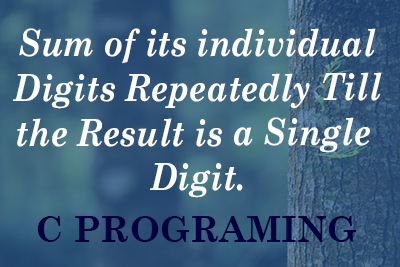Sum of its Individual Digit Until Single Digits - WBUT MCA 2011
18-11-2019    170 times### Write a program to accept a number and find sum of its individual digits repeatedly till the result is a single digit. [WBUT MCA 2011]

```#include <stdio.h>
int main()
{
int no,i,sum;
printf("Enter A Number : ");
scanf("%d",&no);
do{
sum=0;
while(no>0)
{
sum += no%10;
no = no/10;
}
no=sum;
}while(sum>9);
printf("One Digit Sum is %d",sum);
}
```